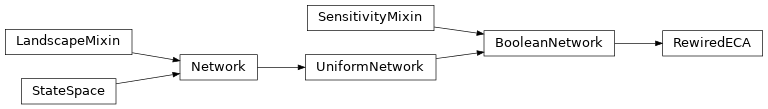# Rewired Elementary Cellular Automata¶

class neet.boolean.RewiredECA(code, boundary=None, size=None, wiring=None, names=None, metadata=None)[source]

RewiredECA represents elementary cellular automaton rule with a rewired topology. That is, RewiredECA is a variant of an neet.boolean.ECA wherein the neighbors of a given cell can be specified by the user. This allows one to study, for example, the role of topology in the dynamics of a network. Every neet.boolean.ECA can be represented as a RewiredECA with standard wiring, but all RewiredECA are fixed sized networks. For this reason, RewiredECA does not derive from neet.boolean.ECA.RewiredECA instances can be instantiated by providing an ECA rule code, and either the number of nodes in the network (size) or a wiring matrix which specifies how the nodes are wired. Optionally, the user can specify boundary conditions as in neet.boolean.ECA. As with all neet.Network classes, the names of the nodes and network-wide metadata can be provided.

In addition to all inherited methods, RewiredECA exposes the following properites

 code The Wolfram code of the elementary cellular automaton boundary The boundary conditions of the elemenary cellular automaton wiring The wiring matrix for the rule.

Examples

If wiring is not provided, the network is wired as a standard neet.boolean.ECA.

>>> reca = RewiredECA(30, size=5)
>>> reca.code
30
>>> reca.size
5
>>> reca.wiring
array([[-1,  0,  1,  2,  3],
[ 0,  1,  2,  3,  4],
[ 1,  2,  3,  4,  5]])


Wiring matrices are $$3 imes N$$ matrices where each column is a node of the network, and the rows represent the left-, middle- and right-input for the nodes. The number of nodes will be inferred from the width of the matrix. For example:

>>> reca = RewiredECA(30, wiring=[[0,1,2],[-1,0,0],[2,3,1]])
>>> reca.code
30
>>> reca.size
3
>>> reca.wiring
array([[ 0,  1,  2],
[-1,  0,  0],
[ 2,  3,  1]])


Here the $$0$$, $$-1$$ and $$2$$ as left, middle and right input. Note that -1 represents the left-boundary condition of the RewiredECA. If instance has periodic boundary conditions then -1 is effectively N-1. Similarly N is the right boundary condition.

To see how the wiring affects the result:

>>> ca = RewiredECA(30, size=3)
>>> ca.update([0, 1, 0])
[1, 1, 1]
>>> ca = RewiredECA(30, wiring=[[0,1,3], [1,1,1], [2,1,2]])
>>> ca.update([0, 1, 0])
[1, 0, 1]

Parameters: code (int) – the 8-bit Wolfram code for the rule boundary (tuple, None) – the boundary conditions for the CA size (int or None) – the number of cells in the lattice wiring (list, numpy.ndarray) – a wiring matrix names (seq) – an iterable object of the names of the nodes in the network metadata (dict) – metadata dictionary for the network ValueError – if both size and wiring are provided ValueError – if neither size nor wiring are provided ValueError – if size is less than $$1$$ (when provided) ValueError – if wiring is not a $$3 \times N$$ matrix (when provided) ValueError – if any element of wiring is outside the range $$[-1,$$] (when provided)
code

The Wolfram code of the elementary cellular automaton

Examples

>>> reca = RewiredECA(30, size=55)
>>> reca.code
30
>>> reca.code = 45
>>> reca.code
45
>>> reca.code = 256
Traceback (most recent call last):
...
ValueError: invalid ECA code

Type: int ValueError – if code is not in $$\{0,1,\ldots,255\}$$
boundary

The boundary conditions of the elemenary cellular automaton

Examples

>>> reca = RewiredECA(30, size=5)
>>> reca.boundary
>>> reca.boundary = (0,1)
>>> reca.boundary
(0, 1)
>>> reca.boundary = None
>>> reca.boundary
>>> reca.boundary = [0,1]
Traceback (most recent call last):
...
TypeError: ECA boundary are neither None nor a tuple

Type: tuple, None ValueError – if boundary is neither None nor a pair of binary states
wiring

The wiring matrix for the rule.

Examples

>>> reca = RewiredECA(30, size=4)
>>> reca.wiring
array([[-1,  0,  1,  2],
[ 0,  1,  2,  3],
[ 1,  2,  3,  4]])
>>> eca = RewiredECA(30, wiring=[[0,1],[1,1],[-1,-1]])
>>> eca.wiring
array([[ 0,  1],
[ 1,  1],
[-1, -1]])
`
Type: numpy.ndarray